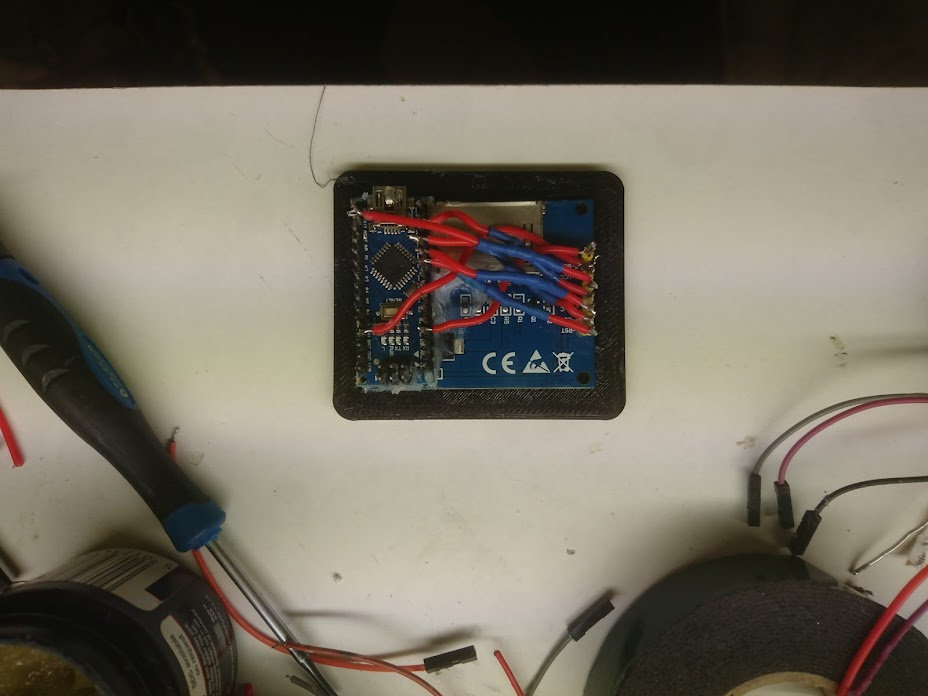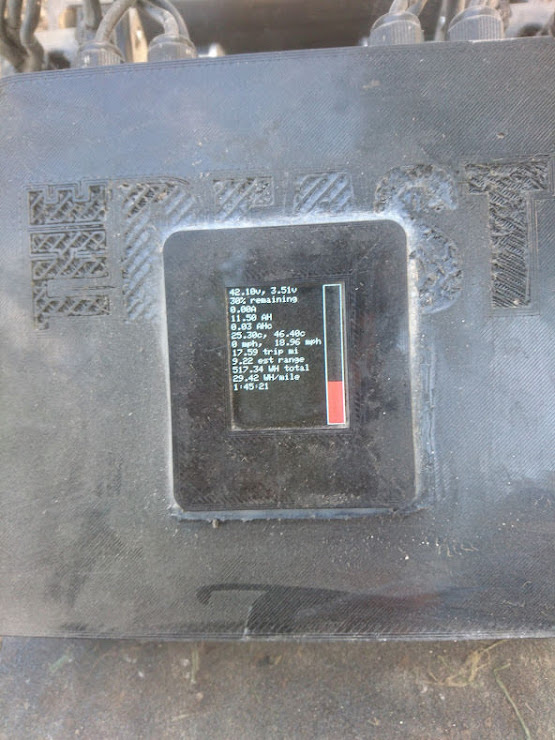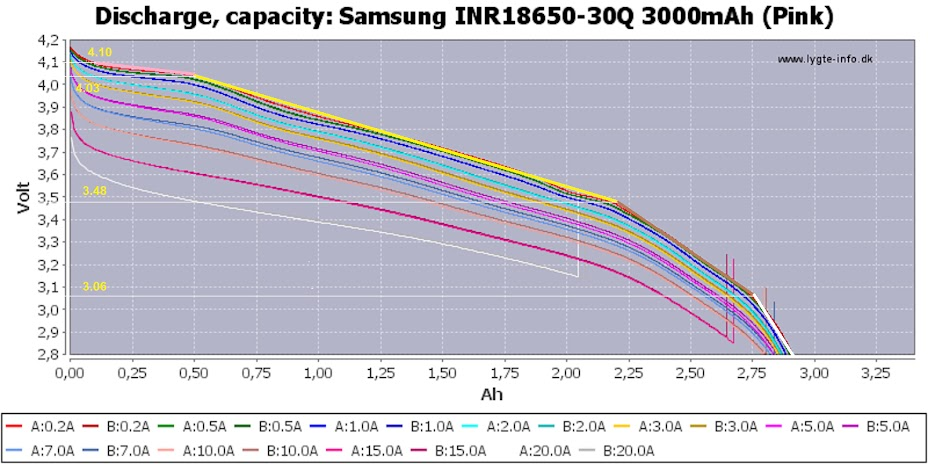# Making an accurate battery meter

Until now ive been using a generic off the shelf battery meter. Which for the Samsung 30Q is highly inaccurate. It reads 17% when the actual level is 30%, it then becomes increasingly inaccurate.

The Vesc has lots of data waiting to be read via serial including the battery input voltage. Many apps take advantage of this but there is no substitute for an on board gauge, plus i could add other useful features such as low battery a warning alarm, speedometer, odometer, range readout etc etc.

The obvious answer was a pi zero or arduino nano with some kind of LCD panel. So i bought cheapest nano and LCD i could find to make a working prototype, with the intent of increasing is functionality once I am happy with the code.

Hooking the arduino up to the LCD was easy enough, make sure you add resistors to improve quality they reduce the voltage fr the LCD the screen looks ans d performs much better at 3.3v.rst D7
CS D9
D/C D8
DIN D11
CLK D13
VCC 5V
BL 5V
GND GND

The code for connecting to the vesc uart was also easy enough using the lastest firmware. It wouldnt work on version 3.40 for me.

The discharge curves for the samung 30q are easy to find, but how to covert t this to code. Manually writing 100 if else statements for each percentage point would take forever. So instead, i broke to curve down into a series of 5 linear lines.The discharge curve is different depending on the current flow through the cell. My favoured method for checking battery level on a ride is stopping mid journey for a few minutes and then checking the resting voltage, so i plotted the red line. My pack is a 7p configuration and my average draw is 15a or 2a per cell ( the turquoise line. The finishing point for this line is very close to the minimal load red line. So my method is pretty reliable.

Another option would be to plot all of the lines and have the meter switch between curves depending on the current readout from the vesc. This means your level readout would be accurate even when riding. Lets stick with method one for now, the math for the curves is the same.

Here is what i came up with. First draw some lines on your batteries discharge curves that best fit the curve. Figure out the start and end voltages of each curve, and the percentage of charge they represent. For my lines they are as follows.

line 1 2900mah to 2500mah remaining, 97% to 83%, 97-83 = 14 percent of the batteries capacity.

line 2 2500mah remaining to 800mah remaining or 83% to 27%, 56 percent of the batteries capacity.

line 3 800mah remaining to 250mah remaining or 27% to 8%, 19 percent of the batteries capacity.

line 4 250mah remaining to 100mah remaining or 8% to 3%, 5 percent of the batteries capacity.

Next the method to calculate the state of charge for each line on the discharge curve.

For example, for line 2.

1. Subtract end of line cell voltage from start of the line cell voltage. 4.03 -3.48 = 0.55v This means from the start to the end of the line the voltage difference is 0.55v.
2. Divide this number the number of percent points the line represents. Line two covers 56% of the batteries charge, so 0.55v / 56 = 0.0098v . This means every drop 0f 0.0098v along this line represents a drop of 1% of the batteries charge.
3. To figure of the batteries state of charge we take the voltage reading from the vesc for example 44.4v, divide is by the number of cells, 12 to get the per cell voltage 44.4v / 12 = 3.7v. We know 3.48v represents 27% so subtract the end of the line voltage from this to get the remainder, 3.7 – 3.48 = 0.22v, now we need to find out what the 0.22v remainer voltage represents.
4. Divide this remaining voltage by the voltage per percentage point we calculated earlier to get the number of percentage points. 0.22v / 0.0098v = 22.5% percent.
5. Finally at this percentage to the end of the line percentage 22.5% + 27% = 49.5% The answer is 44.4v means there is 49.5% charge remaining.

The function to do this for all lines is as follows

``````float volttopercentage(float voltage)
{
float cellvoltage = voltage / 12;

if( cellvoltage > 4.10){
return 100;
}
else if((cellvoltage <4.11)&&( cellvoltage > 4.03 )){

cellvoltage = cellvoltage - 4.03;
percentage = cellvoltage / 0.0050;
percentage = percentage + 83;
return percentage;
}
else if((cellvoltage <4.031)&&( cellvoltage > 3.48 )){
cellvoltage = cellvoltage - 3.48;
percentage = cellvoltage / 0.0098;
percentage = percentage + 27;
return percentage;
}
else if((cellvoltage <3.481)&&( cellvoltage > 3.06 )){
cellvoltage = cellvoltage - 3.06;
percentage = cellvoltage / 0.0221;
percentage = percentage + 8;
return percentage;
}
else{
cellvoltage = cellvoltage - 2.80;
percentage = cellvoltage / 0.052;
percentage = percentage + 3;
return percentage;
//sound alarm
}
}``````

Ill release the full code here shortly. Im using the developmental version of solid geeks library